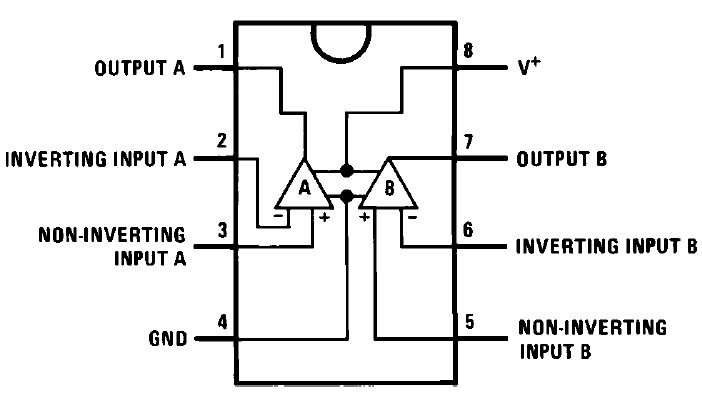### Over-voltage and Under-voltage protection Circuit for  High and Low Voltage Cutoff.

This voltage sensor circuit can be used to protects the any appliance to voltage fluctuation . When input voltage goes high then the relay will activated and also when the input voltage is below then required level then relay activated.

Note- Load is connected with NC and COM pin of Relay

Dual comparator ic LM358 is used to make this circuit diagram. Here both two comparators of 358 ic is used. Actually AC supply is not detected by the circuit  , Circuit is detected AC supply through DC converter. So we need to DC power for circuit operation. When AC supply is fluctuate high and low then the circuit is also get a variable DC power and then circuit is able to sense the voltage condition.

When the input voltage is greater than the reference voltage then the output of comparator is himechagh and if input voltage is lower than reference voltage then the output is LOW. This mechanism of comparator is used in this circuit to sense and compare the input supply voltage.

Reference voltage means the input at inverting pin (pin 2 & 6 ). Here voltage of inverting input pin is fixed 5.1v by zener diode. You can choose other value by different zener diode below the value of input voltage.

At the normal voltage level. The output of comparator is LOW because of the input voltage is lower than reference voltage, And relay is not activated , Appliance are ON. IF AC voltage increase then the input voltage at pin 3 is also increased. As When the input voltage goes high then reference voltage at pin 2 , The Relay is activate and pin of relay is changed from NC to NO and connected load appliance is disconnected.

In this circuit reference voltage is 4v. so as soon input voltage go more than 4v then the relay is activated.

Now,

In 2nd Comparator (U2B) the Zener Diode is connected with non  inverting input .

Assume that the AC supply is decreased , when ac supply goes LOW, then the Reference voltage of comparator 2 is also get decreased as compare to the input voltage . Input voltage is fixed by a 4v zener diode. so as reference voltage is decreased below the input voltage then the output of comparator goes HIGH. Relay is activated again andConnected load is disconnected and turned off.

So Output Of comparator is LOW And Load Get Supply voltage - If only the input voltage Of comparator 1 is below the 5.1v. And the reference voltage of comparator 2 is more than 4v. The required cutoff level is set by adjusting the preset.

If a comarator output is HIGH and other Comparator output is LOW then Only High signal is passed and relay get only high signal , No LOW/Negative signal because base of transistor is connected through a Diode.

so this is a protection circuit with high and low voltage. You can use 6.8v and 6.0v zener diode. And can useLM324 opamp Comparator ic for this circuit.

Vin> Vref= High Output (+vcc output)

Vin<Vref = LOW Output (-ve vcc output)     Vin = input voltage , Vref= reference voltage

Adjust the cutoff level by the preset adjustment.

Components

IC- LM358-1

Zener Diode

5.1v -1

4v-1

Resistor

5k-2

10k-2

33k-1

1k-2

2.2k-1

Preset-50k-2

capacitor

0.1uf-1

1000uf 35v-1

Diode- 5

Relay 12v-1

7812 regulator ic-1

transformer -12v 250ma-1

Transistor BC547-1

LED-1